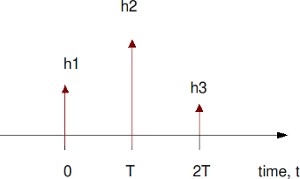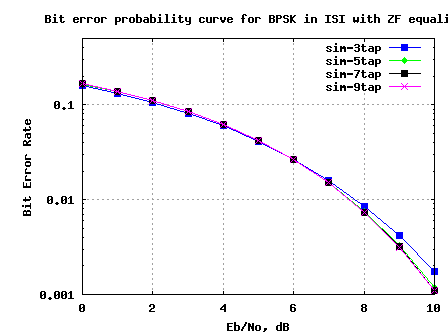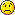(4 votes, average: 5.00 out of 5)Loading ...

# BER for BPSK in ISI channel with Zero Forcing equalization

by on November 29, 2009

In the past, we had discussed BER for BPSK in flat fading Rayleigh channel. In this post, lets discuss a frequency selective channel with the use of Zero Forcing (ZF) equalization to compensate for the inter symbol interference (ISI). For simplifying the discussion, we will assume that there is no pulse shaping at the transmitter. The ISI channel is assumed to be a fixed 3 tap channel.

## Transmit symbol

Let the transmit symbols be modeled as

, where

is the symbol period,

is the symbol to transmit,

is the transmit filter,

is the symbol index and

is the output waveform.

For simplicity, lets assume that the transmit pulse shaping filter is not present, i.e .

So the transmit symbols can be modeled by the discrete time equivalentFigure: Transmit symbols

## Channel Model

Lets us assume the channel to be a 3 tap multipath channel with spacing i.e.Figure: Channel model (3 tap multipath)

In addition to the multipath channel, the received signal gets corrupted by noise , typically referred to as Additive White Gaussian Noise (AWGN). The values of the noise follows the Gaussian probability distribution function, with

mean and

variance .

The received signal is

, where

is the convolution operator.

## Zero Forcing Equalization

Objective of Zero Forcing Equalization is to find a set of filter coefficients which can make .

After equalization

.

Note:

The term causes noise amplification resulting poorer bit error rate performance.

Deriving the equalization coefficients

From the post on toeplitz matrix,we know that convolution operation can be represented as matrix multiplication.

% Matlab code for using Toeplitz matrix for convolution
clear all
x = [1:3];
h = [4:6];
xM = toeplitz([x zeros(1,length(h)-1) ], [x(1) zeros(1,length(h)-1) ]);
y1 = xM*h';
y2 = conv(x,h);
diff = y1'-y2
diff = [  0   0   0   0   0 ]

Using similar matrix algebra and assuming that the coefficients has 3 taps, the equation can be equivalently represented as,

Solving for , we have

.

If we assume that has 5 taps,

Solving for , we have

.

Example

% Assuming a 3 tap channel as follows
ht = [0.2 0.9 0.3];
L = length(ht);
kk = 1;
hM = toeplitz([ht([2:end]) zeros(1,2*kk+1-L+1)], [ ht([2:-1:1]) zeros(1,2*kk+1-L+1) ]);
d  = zeros(1,2*kk+1);
d(kk+1) = 1;
c  = [inv(hM)*d.'].';

The frequency response of the channel and the equalizer are shown below:Figure: Frequency response of the channel and the equalizer

## Simulation Model

The attached Matlab/Octave simulation script performs the following:

(a) Generation of random binary sequence

(b) BPSK modulation i.e bit 0 represented as -1 and bit 1 represented as +1

(c) Convolving the symbols with a 3-tap fixed fading channel.

(d) Adding White Gaussian Noise

(e) Computing the equalization filter at the receiver – the equalization filter is 3, 5, 7, 9 taps in length

(f) Demodulation and conversion to bits

(g) Counting the number of bit errors

(h) Repeating for multiple values of Eb/No

The simulation results are as shown in the plot below.Figure: BER plot for BPSK in a 3 tap ISI channel with Zero Forcing equalizer

Observations

1. Increasing the equalizer tap length from 3 to 5 showed reasonable performance improvement.

2. Diminishing returns from improving the equalizer tap length above 5.

3. The results are poorer compared to the AWGN no multipath results. This is due to the noise amplification (see the frequency response above) by the zero forcing equalization filter.

Next step is to discuss the zero forcing equalizer in the presence of transmit pulse shaping and then move on to minimum mean square error equalizer.

D id you like this article? Make sure that you do not miss a new article by subscribing to RSS feed OR subscribing to e-mail newsletter. Note: Subscribing via e-mail entitles you to download the free e-Book on BER of BPSK/QPSK/16QAM/16PSK in AWGN.

{ 56 comments… read them below or add one }chayanika November 17, 2012 at 8:23 pm

sir,

i m doing a project on interference mitigation in UWB channel. I hav choose transmitted reference receiver to mitigate interference. hav not able to model the channel..:-( m i proceeding in a right way??

plz sir help me…Krishna Sankar November 18, 2012 at 6:30 am

@chayanika: For modeling the channel, one can start of with a simple AWGN noise model and later one can add multipath to it. For modeling interference, one need to idea of how is interfering – Is it another UWB transmitter operating in the same frequency?Dibyani November 5, 2012 at 8:50 am

Have you developed any code for co-channel interfernce in MIMO channelKrishna Sankar November 12, 2012 at 7:05 am

@Dibyani: sorry, noAmire October 6, 2012 at 2:24 am

hello mr
realllllllllllllllly Very nice work sir!!

can you help me for use that in mimo channel (alamouti method)?

how shoul I do in combiner level?I should use conv ? or multiply?(

plz help me I am really need it :’(Krishna Sankar October 6, 2012 at 6:41 am

@Amire: Typically, when dealing with MIMO channel (especially using OFDM), it is a convolution in the time domain. However, assuming that the channel is contained with in the cyclic prefix, the effect of channel in frequency domain is a single mutliplier with amplitude/phase values.mepal June 5, 2012 at 11:40 pm

My question is related to MF part.
If we perform pulse shaping g(t) at the transmitter then the overall pulse shape is g(t)*h(t) and the received waveform would be

r(t) = a_k( g(t)*h(t) ) + n(t)

at the receiver the MF would be matched to (g(t)*h(t)) followed by a sampler and LE.

but in this MATLAB CODE setting the , the first thing i see is LE……(which is confusing.)
followed by another convolution (about which you have commented that its there to take care of pulse shaping done at TX which does not exist in this example and is therefore redundant)

can you plz explain this.Krishna Sankar June 11, 2012 at 5:19 am

@mepal:
Thanks for pointing that out. In this simulation, have not used any pulse shaping. The code which has the comment stating it is matched filtering is indeed doing the equalization part.

Another comment which I have is : if we have inter symbol interference, then it is preferable to do equalization first and then do the matched filtering. Agree?Sylva May 3, 2012 at 8:06 pm

Hi,
Please I need some explanation on the first two lines of the matches filter part;specifically,why you used kk+2

yFilt = yFilt(kk+2:end);

Thanks.Krishna Sankar May 15, 2012 at 6:00 am

@Sylva: The kk+2 is to ignore the first few samples caused by the delay of the filter.Siva April 3, 2012 at 6:46 am

Hi,

Do you have the theoritical BER for zero forcing equalization and MMSE equalization?

Thanks
SivaKrishna Sankar April 5, 2012 at 5:17 am

@Siva: Hmm.. noBinh March 9, 2012 at 6:59 pm

Hi Krishna Sankar,

Can you explain a little bit more about the ‘Matched Filter’ part?
yFilt = yFilt(kk+2:end);
yFilt = conv(yFilt,ones(1,1)); % convolution
ySamp = yFilt(1:1:N); % sampling at time T
Thank you.Krishna Sankar March 12, 2012 at 4:50 am

@Binh: Don’t think we need the matched filter part given that we have not put any pulse shaping in the transmitter section. May have been inherited from some existing code…Siva March 1, 2012 at 6:47 am

Could you please let me know the purpose of this step? Why are we performing convolution with 1 at the receiver?

yFilt = conv(y,c);
yFilt1 = yFilt(kk+2:end);
yFilt = conv(yFilt,ones(1,1)); % convolution
ySamp = yFilt(1:1:N); % sampling at time T

Thanks.Krishna Sankar March 12, 2012 at 5:01 am

@Siva: The last two line may have been inherited from existing code which had pulse shaping in the transmitter. Sorry about that.Fantaye May 14, 2011 at 2:02 am

Thank you a lot all are really help full!

I will be happy if any one help me using LMS for MIMO systemsKrishna Sankar May 24, 2011 at 5:17 am

@Fantaye: Guess, you want to use LMS for channel tracking?vsk June 26, 2010 at 2:16 am

Hi Mr.Krishna,
For a MIMO system, how do I model an ISI channel? Meaning do I sum the impulse responses for the various paths from antenna i to antenna j and denote it as a single co efficient in the H matrix as h(ji)?
And so how do I perform ZF-SIC for the same case?Krishna Sankar June 28, 2010 at 6:17 am

@vsk: In a MIMO ISI case, convolve each transmit stream with the channel seen from each transmit stream to receive antenna.
For eg, if
h11 – channel between transmit antenna 1 to receive antenna 1
h12 – channel between transmit antenna 2 to receive antenna 1
h21 – channel between transmit antenna 1 to receive antenna 2
h22 – channel between transmit antenna 2 to receive antenna 2

The received symbol on receive antenna 1
y1 = conv(h11,x1) + conv(h12,x2) + n1
y2 = conv(h21,x1) + conv(h22,x2) + n2
and x1, x2 are transmit symbols from antenna 1 and 2 respectively.

Do NOT sum all the impulse response and represent them as a single value.Ali April 15, 2010 at 9:33 pm

Hi i have a question regarding the ZF-equlization, what if the desired tap is at the last position?
h = [0.3 0.1 0.9]. I tried to change the code but i could not.Krishna Sankar April 18, 2010 at 2:16 pm

@Ali: Try changing the creation of the Toeplitz matrix such that h3 comes in the diagonal term…mepal September 21, 2012 at 3:06 am

Can you please explain why hM

hM = toeplitz([ht([2:end]) zeros(1,2*kk+1-L+1)], [ ht([2:-1:1]) zeros(1,2*kk+1-L+1) ])
for kk=1 is

hM =

0.9000 0.2000 0
0.3000 0.9000 0.2000
0 0.3000 0.9000

0.2000 0 0
0.9000 0.2000 0
0.3000 0.9000 0.2000
0 0.3000 0.9000
0 0 0.3000

given h0=0.2 , h1=0.9 , h2=0.3

when we perform convolution one of the signals is flipped at t=0.
this means that the flipped signal should be

h(-2) = 0.3
h(-1) = 0.9
h(0) = 0.2

so the convolution matrix should look like the second matrix.

(i used convmtx(ht’,2*kk+1) to generate the 2nd matrix)

kindly let me know what point i m missing here.Krishna Sankar September 22, 2012 at 6:04 am

@mepal: the end goal is to make conv(c,ht) = impulse.mepal September 22, 2012 at 9:53 pm

i have one more question here:

we want to make the cascade of channel and equalizer equivalent to a single impulse(incase of ZF LE).
How is this different from Partial Response Target Equalization (PRTE).

In PRTE one defines a target and derive optimal equalizer by minimizing MSE. So there is an optimality criterion involved in the derivation of PTRE but apart from that we desire to have the conv(c,ht)=target .

Can we comment that MMSE LE is same as PTRE (both r derived based on minimizing MSE)?
Is the above observation correct?Newbie April 14, 2010 at 7:58 pm

Hiii Mr.Krishna

Thank you for ur post, that is help me so much. That is time invariant channel???Krishna Sankar April 18, 2010 at 2:11 pm

@Newbie: A channel whose taps do not vary with time is a time invariant channelAhmed February 18, 2010 at 12:29 pm

Hi Krishna,
One question about the channel coeffecients. They are real number as I we find here. But in case of Rayleigh channel they are complex where both real are imaginary parts are samples from Gaussain distribution. My question is if we consider a static ISI channel with complex coeffcients, is there anything wrong with it?Since the co-efficients refer to the gain of each tap, I feel complex values are also possible where the imaginary parts refers to the phase.
Waiting for your response.
AhmedKrishna Sankar March 31, 2010 at 5:41 am

@Ahmed: Complex channel coefficients are also possibleIshwinder February 11, 2010 at 7:28 am

Hi Krishna,

Request you to please explain the below mentioned part of your code.

K=4; What is the significance of this K and why is it equal to 4?

for kk = 1:K

L = length(ht);
hM = toeplitz([ht([2:end]) zeros(1,2*kk+1-L+1)], [ ht([2:-1:1]) zeros(1,2*kk+1-L+1) ]);
d = zeros(1,2*kk+1);
d(kk+1) = 1;
c = [inv(hM)*d.'].’;

% mathched filter
yFilt = conv(y,c);
yFilt = yFilt(kk+2:end);
yFilt = conv(yFilt,ones(1,1)); % convolution
ySamp = yFilt(1:1:N); % sampling at time T

Can we not perform the above operations using just conv or filter functions in Matlab?

Thanks
IshwinderLoïc February 26, 2010 at 12:27 am

K = 4 equalizers. Inside the loop, the length of the equalizer is chosen by 2*kk+1, where kk = 1:4 (3,5,7,9).Krishna Sankar March 30, 2010 at 5:43 am

@Loic: hmm… okKrishna Sankar April 4, 2010 at 4:04 am

@Ishwinder: I wanted to compare 3tap/5tap/7tap/9tap equalization case
k = 1 –> 3 tap; k = 2–> 5 tap and so onAndrew February 2, 2010 at 5:40 pm

hello, Krishna Sankar, I have a question on ZF for ISI channel.
As your example, I have ISI channel with three taps [h1 h2 h3], then the signal [S1 S2 S3 S4 S5 S6 S7 ...] passes through the channel. At the receiver, the received signal denoted by [R1 R2 R3 R4 R5 R6 R7...]. For example, I want to detect symbol R6, we can readily find that R6=h1*S6+h2*S5+h3*S4, then this signal passes through ZF equalizer with coefficients [C1 C2 C3]. Then the estimated symbol for R6 will be :
^R6=C1*R6+C2*R5+C3*R4=C1h1S6+(C1h2+C1h1)S5+(C1h3+C2h2+C3h1)S4+(C2h3+C3h2)S3+c3h3S2

Why it’s different from your derivation results. Can you tell where I’m wrong.Krishna Sankar April 4, 2010 at 4:28 am

@Andrew: Its a convolution operation.j.ravindra babu February 2, 2010 at 1:20 pm

please send me multi-user detectors in ds-cdmaKrishna Sankar April 4, 2010 at 4:28 am

@j.ravindra babu: Sorry, I do not knowCHANDU March 14, 2013 at 11:05 am

hi sir
plz help on code for multiuser detection in cdmaKrishna Sankar March 15, 2013 at 5:24 am

@CHANDU: In CDMA, each user is assigned a code. Run the received samples through a filter matched to each codeAnanthi January 28, 2010 at 3:12 pm

It’s a useful one for meKrishna Sankar April 4, 2010 at 4:50 am

@Ananthi: Thanksrajesh neelakandan January 27, 2010 at 8:08 pm

Thanks for your post!

When I saw some old posts, many red “X” appear where the formula or symbol should be. How can I solve it ? Is something wrong with my browser?Krishna Sankar January 28, 2010 at 5:22 am

@rajesh: Can you please list the posts where you saw this error. This is not expected. Anyhow once you give the list, I will take a look.Alif January 12, 2010 at 7:21 am

hi,
ht = [0.4 0.9 0.3];
I tried another value like ht = [0.6 0.9 0.3];
then BER increased dramatically, it doesn;t make sense
Can you tell me what’s the problem? THXCronaldo January 3, 2010 at 11:09 am

Thanks for your post!

When I saw some old posts, many red “X” appear where the formula or symbol should be. How can I solve it ? Is something wrong with my browser?Sohbet December 10, 2009 at 4:16 am

Thanks A Lot For ThisPuripong December 7, 2009 at 8:37 am

Hi Krishna

Thank you for very nice post

May I ask you about the basic question

From the script below
10^(-Eb_N0_dB(ii)/20)*n; % additive white gaussian noise

I know that
10log10(A_power) = A_power dB —- (1)
20log10(A_voltage) = A_voltage dB —– (2)

when we try to convert Eb_N0 into dB we use formula (1)
10log10(Eb_N0) = Eb_N0_dB

Why does the division factor equal to 20 ?

ThanksKrishna Sankar December 8, 2009 at 5:24 am

@Puripong: We want to convert scale the noise voltage by a factor corresponding to Eb_N0_dB. To convert dB to voltage we have the 1/20 term (as you have pointed out in (2)). Helps?Puripong December 8, 2009 at 7:45 am

You mean….
We want to convert Eb_N0_dB into noise voltage.
sqrt(voltage) = Eb_N0_dB
voltage = Eb_N0_dB^2
10log10(Eb_N0_dB^2) = 20log10(Eb_N0_dB)
So, we have the division factor equal to 20

Ohhh, Surely I missed.
Thank you very muchKrishna Sankar December 10, 2009 at 5:32 am

@Puripong: Glad to helpAyhem December 3, 2009 at 1:18 am

Very nice work , AMAZING

Keep GoningKrishna Sankar December 7, 2009 at 5:07 am

@Ayhem: ThanksCommunications Engineer December 1, 2009 at 8:15 pm

Fantastic work! really. Can’t wait for MMSE equalization (I think that would be next)

Krishna, would you please help me with the two questions I have with respect to your posts on OFDM and EGC. I have provided the link below

I’d appreciate your helpKrishna Sankar December 7, 2009 at 5:03 am

@communications_engineer: Thanks. Hope my reply to both the comments addressed some of your concerns. Good luck.Wig November 30, 2009 at 10:13 am

Wow~~ New topic, I like it~!

thank you so much!!Krishna Sankar September 26, 2012 at 5:35 am